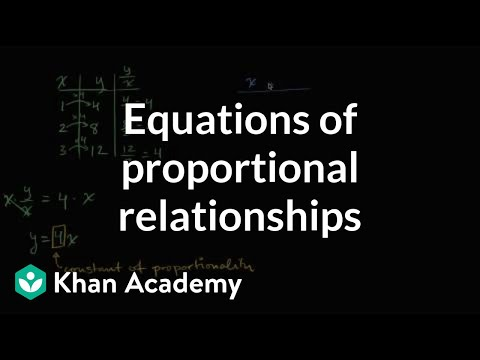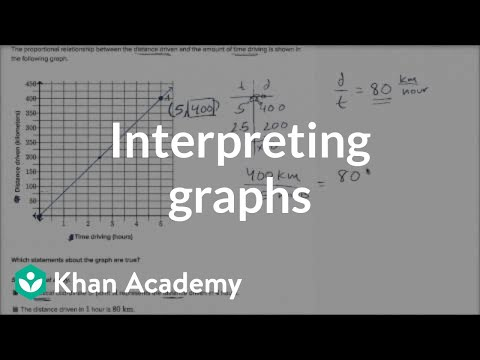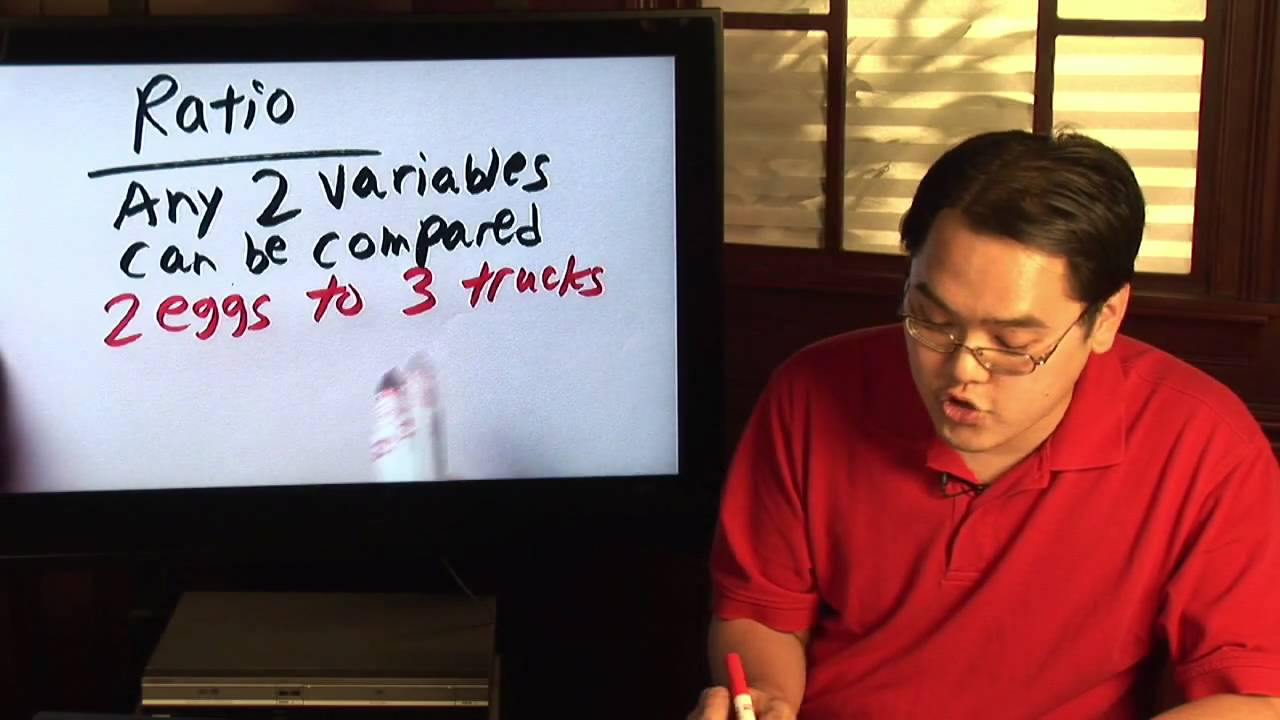# Writing proportional equations

##By Khan Academy

Learn how to write a proportional equation y=kx where k is the so-called constant of proportionality.# Interpreting graphs of proportional relationships

##By Khan Academy

Learn how to read and understand graphs of proportional relationships# Solving an Equation Involving Rational Exponents - Example 3

##By PatrickJMT

YouTube presents Solving an Equation Involving Rational Exponents - Example 3, an educational video resource on math.# Fractions & Proportions : Understanding Math Ratios

##By

In math, ratio is a fraction in which two variables can compared. Discover how to convert ratios into fractions with help from a math teacher in this free vi...# Writing proportional equations

##By Khan Academy

Learn how to write a proportional equation y=kx where k is the so-called constant of proportionality.# Writing proportional equations

##By Khan Academy

Learn how to write a proportional equation y=kx where k is the so-called constant of proportionality.# Writing proportional equations

##By Khan Academy

Learn how to write a proportional equation y=kx where k is the so-called constant of proportionality.# Writing proportional equations

##By Khan Academy

Learn how to write a proportional equation y=kx where k is the so-called constant of proportionality.